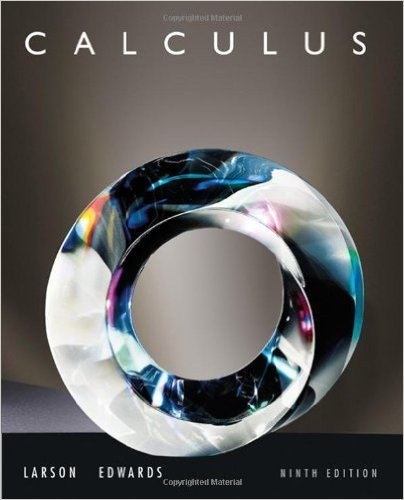×
×

# Solutions for Chapter 15.2: Line Integrals## Full solutions for Calculus | 9th Edition

ISBN: 9780547167022Solutions for Chapter 15.2: Line Integrals

Solutions for Chapter 15.2
4 5 0 409 Reviews
12
2
##### ISBN: 9780547167022

Calculus was written by and is associated to the ISBN: 9780547167022. Chapter 15.2: Line Integrals includes 87 full step-by-step solutions. This textbook survival guide was created for the textbook: Calculus , edition: 9. This expansive textbook survival guide covers the following chapters and their solutions. Since 87 problems in chapter 15.2: Line Integrals have been answered, more than 63101 students have viewed full step-by-step solutions from this chapter.

Key Calculus Terms and definitions covered in this textbook
• Axis of symmetry

See Line of symmetry.

• Bounded interval

An interval that has finite length (does not extend to ? or -?)

• Commutative properties

a + b = b + a ab = ba

• Compounded k times per year

Interest compounded using the formula A = Pa1 + rkbkt where k = 1 is compounded annually, k = 4 is compounded quarterly k = 12 is compounded monthly, etc.

• Dependent variable

Variable representing the range value of a function (usually y)

• Derivative of ƒ at x a

ƒ'(a) = lim x:a ƒ(x) - ƒ(a) x - a provided the limit exists

• Directed distance

See Polar coordinates.

• DMS measure

The measure of an angle in degrees, minutes, and seconds

• Head minus tail (HMT) rule

An arrow with initial point (x1, y1 ) and terminal point (x2, y2) represents the vector <8x 2 - x 1, y2 - y19>

• Logarithmic form

An equation written with logarithms instead of exponents

• Minor axis

The perpendicular bisector of the major axis of an ellipse with endpoints on the ellipse.

• Newton’s law of cooling

T1t2 = Tm + 1T0 - Tm2e-kt

• Odd function

A function whose graph is symmetric about the origin (ƒ(-x) = -ƒ(x) for all x in the domain of f).

• Origin

The number zero on a number line, or the point where the x- and y-axes cross in the Cartesian coordinate system, or the point where the x-, y-, and z-axes cross in Cartesian three-dimensional space

• Permutation

An arrangement of elements of a set, in which order is important.

The graph in three dimensions of a seconddegree equation in three variables.

• Residual

The difference y1 - (ax 1 + b), where (x1, y1)is a point in a scatter plot and y = ax + b is a line that fits the set of data.

• Root of an equation

A solution.

• Row operations

See Elementary row operations.

• Standard form of a polar equation of a conic

r = ke 1 e cos ? or r = ke 1 e sin ? ,

×